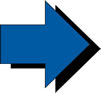The "if" StatementIt's time to make decisions using the relational operators.

The "if" expression is called a conditional statement (decision statement).  It tests a relationship by using the relational operators.  Based upon the results of these comparisons, decisions are made as to which statement(s) the program will execute next.

 if (test condition)   {       block of one;       or more C++ statements; }If you put a semicolon after the test condition, the "if" statement will STOP.  The block will not be executed.

The test condition can be any relational comparison (or logically TRUE) statement and must be enclosed in parentheses.  The block of statements is enclosed in French curly braces and are indented for readability.  The braces are NOT required if only ONE statement follows the "if", but it is a good idea to get in the habit of including them.

The block executes only if the test condition is TRUE.  If the test condition is FALSE, the block is ignored and execution continues to the next statement following the block.

 Be careful!!!    A careless mistake may go unnoticed. if (a = 3)  is almost ALWAYS true.  The condition a = 3 is actually an "assignment statement" (most likely NOT what you really intended to type), and assignments are consider to be true for any value other than 0.  If the assignment value is 0, such as if (a = 0), the condition is considered false. whereas: if (a = = 3)   is true ONLY if the value stored in a is the number 3.  This is most likely what you intended to type.

Examples:

 // using a character literal if (grade = = 'A') {      cout<<"Put it on the frig!"; } // logically true test condition - // when x is a non-zero value the // test condition is considered true if (x) {      cout<<"Way to go!";      cout<<"The x is not zero!"; } // using two variables if (enemyCount < heroCount) {      cout<<"The good guys win!"; } // using a calculation if (cost * number = = paycheck) {      inventory = 0; }